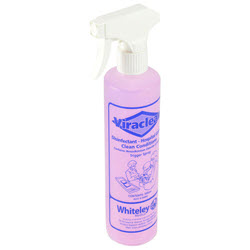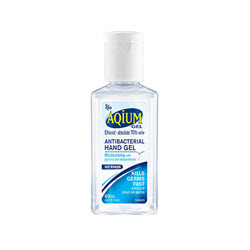# Hygiene / Disinfectant

Grid List
\$31.33
Increase value Decrease value
\$18.77
Increase value Decrease value
\$21.74
Increase value Decrease value
\$108.79
Increase value Decrease value
\$13.68
Increase value Decrease value
\$44.25
Increase value Decrease value
\$39.23
Increase value Decrease value
\$24.78
Increase value Decrease value
\$19.75
Increase value Decrease value
\$12.77
Increase value Decrease value
\$25.72
Increase value Decrease value
\$16.81
Increase value Decrease value
\$22.00
Increase value Decrease value
\$132.00
Increase value Decrease value
\$194.70
Increase value Decrease value
\$25.41
Increase value Decrease value
\$10.78
Increase value Decrease value\$22.63
Increase value Decrease value
\$17.00
Increase value Decrease value
\$101.00
Increase value Decrease value\$5.68
Increase value Decrease value
\$17.29
Increase value Decrease value
\$81.36
Increase value Decrease value
\$8.71
Increase value Decrease value
\$30.75
Increase value Decrease value
\$36.49
Increase value Decrease value
Grid List
##### Products to compare:
Comparing Products